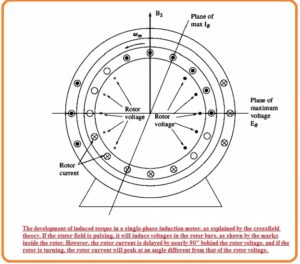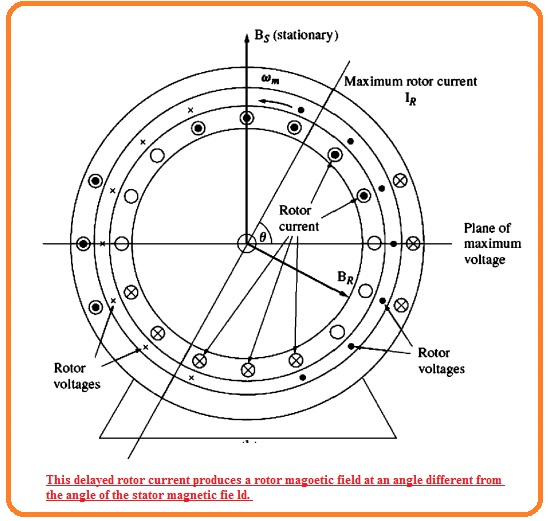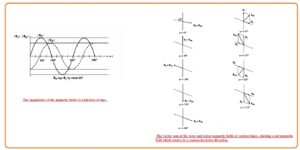Hi, reader welcomes to the new post. In this tutorial, we will discuss the Cross-Field Theory of Single-Phase Induction Motors. The induction motor belongs to the ac family of motors. There are two main types of induction motors single-phase and three-phase induction motor. single motor called single phase motor since it operates on single-phase and three-phase induction motor operates on 3 phases.

In this post, we will cover details about the cross-field theory of single phase induction motor and its related parameters. So let’s get started.

## Cross-Field Theory of Single-Phase Induction Motors

• The cross-field theory of single phase induction motor helps to view the induction motor with a different point of view.
• This theory is related to the voltage and current which are static stator field can produce in the windings of the rotor when the rotor is rotating.
• Let us assume that single-phase induction motor having a rotor that is taken to speed through the use of any exterior technique.
• This type of motor can see here.• Voltage is produced in the rotors through the peak voltage existing in the windings moving directly through the windings existing in the stator.
• This rotor voltage generates a current in the rotor but the sine of the high value of reactance of the rotor, the current lags the voltage through the angle of ninety degrees.
• The rotor rotating speed is almost equal to the synchronous speed so ninety degrees lagging factor in current generates the ninety degrees angular shift among the plane of peak rotor voltage and the plane of peak current.
• In the below figure you can see the resultant rotor field.• The rotor field is less than the stator field, since the loss of rotor but there is difference of ninety degrees angle in space and time as well.
• If these 2 fields are added for different time intervals so we can see that the net field in the motor is revolving in the anticlockwise direction.• Through the rotating field existing in the motor induction motor will create the total torque in the direction of movement and that torque will retain the rotor moving.
• If the rotor of the motor is moving in the clockwise direction the net torque will have clock-wise direction will again retain the motor moving.

That is a detailed post about the cross-field theory of induction motor. If you have any further query ask in the comments. Thanks for reading. Have a nice day